# AI玩微信跳一跳的正确姿势

Published: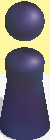def multi_scale_search(pivot, screen, range=0.3, num=10):
H, W = screen.shape[:2]
h, w = pivot.shape[:2]

found = None
for scale in np.linspace(1-range, 1+range, num)[::-1]:
resized = cv2.resize(screen, (int(W * scale), int(H * scale)))
r = W / float(resized.shape)
if resized.shape < h or resized.shape < w:
break
res = cv2.matchTemplate(resized, pivot, cv2.TM_CCOEFF_NORMED)

loc = np.where(res >= res.max())
pos_h, pos_w = list(zip(*loc))

if found is None or res.max() > found[-1]:
found = (pos_h, pos_w, r, res.max())

if found is None: return (0,0,0,0,0)
pos_h, pos_w, r, score = found
start_h, start_w = int(pos_h * r), int(pos_w * r)
end_h, end_w = int((pos_h + h) * r), int((pos_w + w) * r)
return [start_h, start_w, end_h, end_w, score]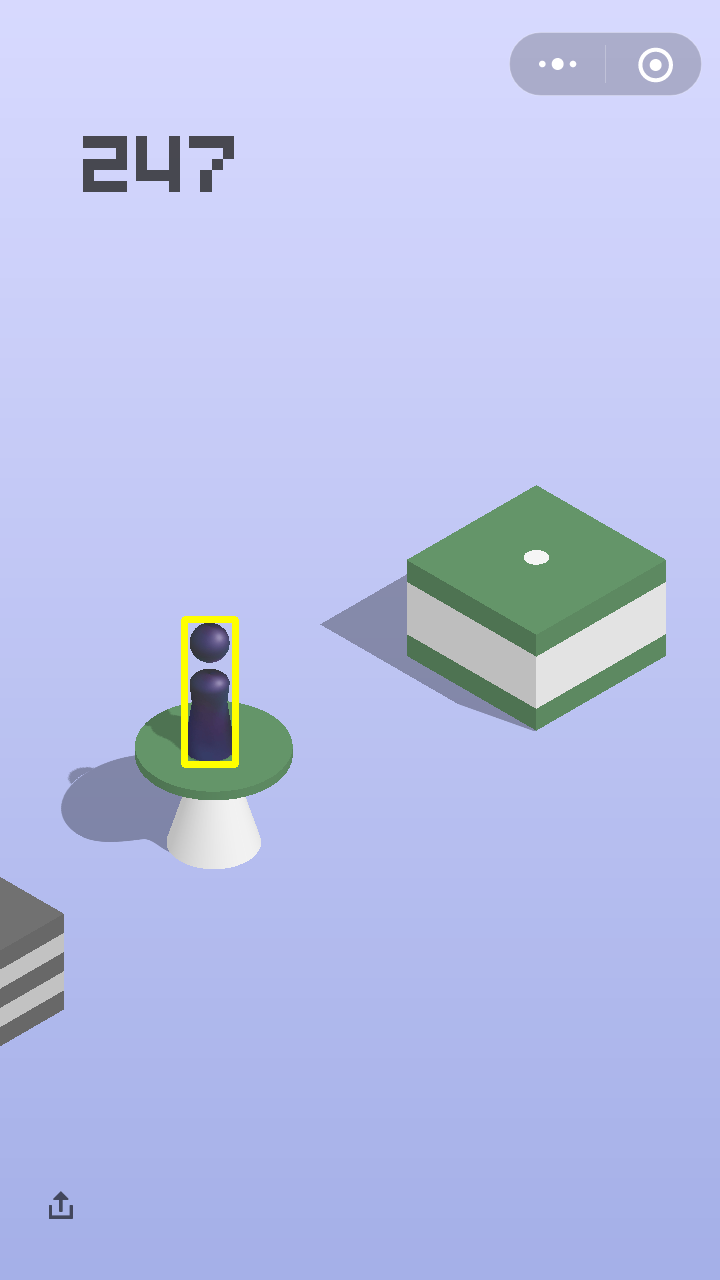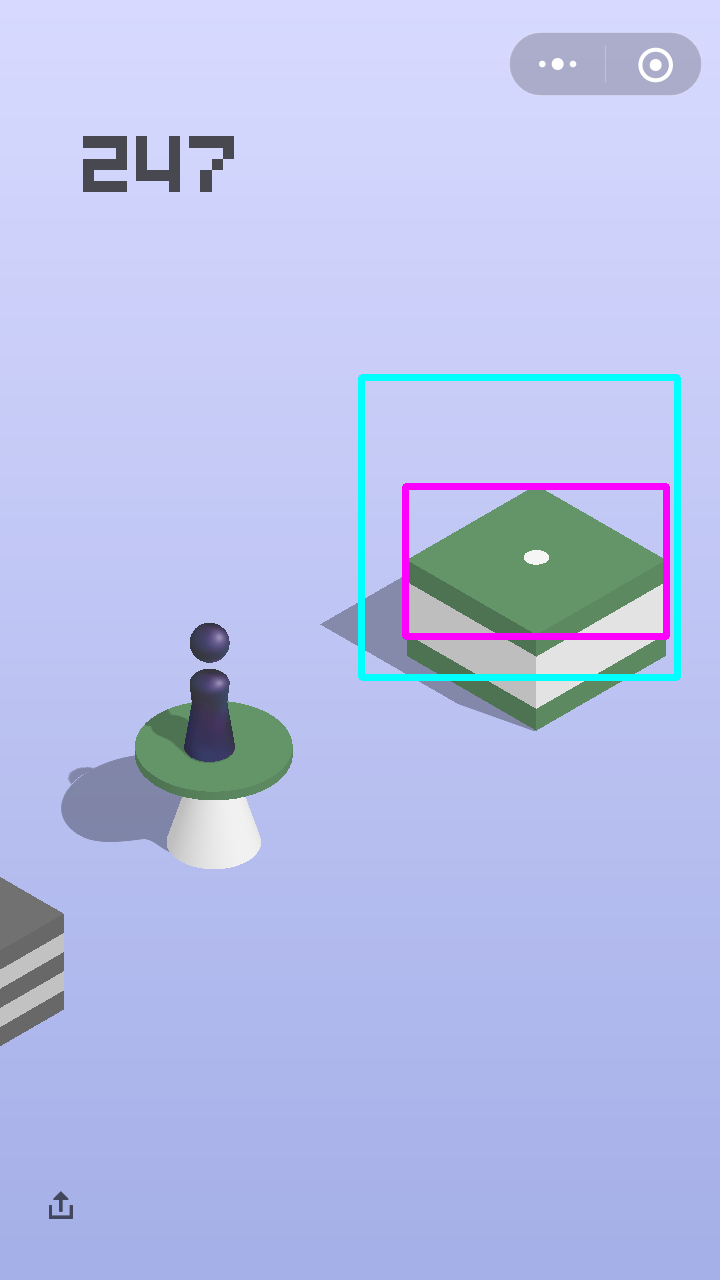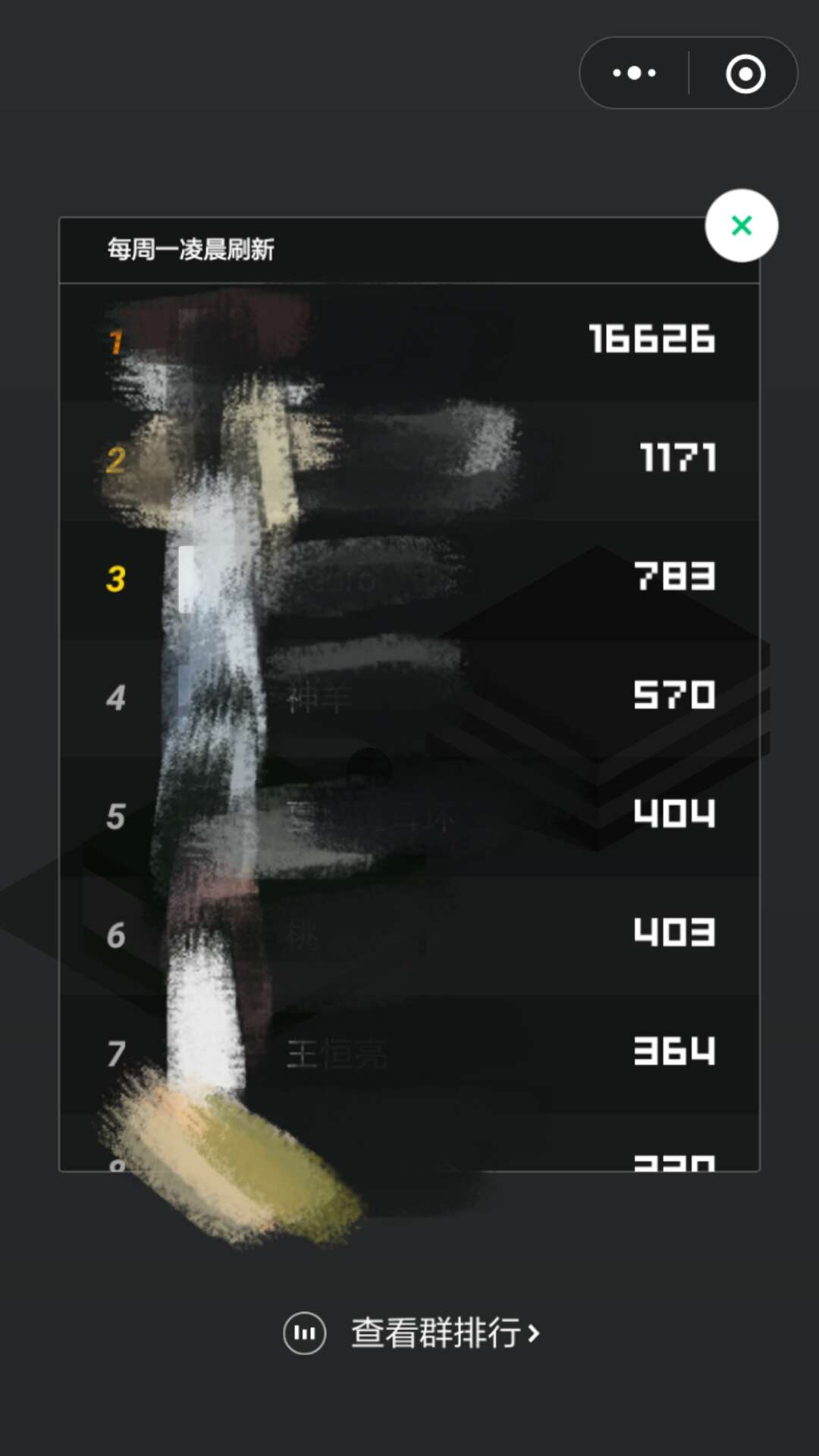### CNN Coarse-to-Fine 模型

#### 数据采集与预处理

##### coarse 模型数据预处理##### fine 模型数据预处理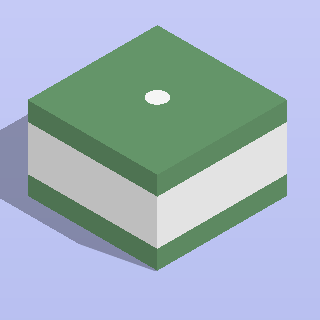#### coarse 模型

def forward(self, img, is_training, keep_prob, name='coarse'):
with tf.name_scope(name):
with tf.variable_scope(name):
out = self.conv2d('conv1', img, [3, 3, self.input_channle, 16], 2)
# out = tf.layers.batch_normalization(out, name='bn1', training=is_training)
out = tf.nn.relu(out, name='relu1')

out = self.make_conv_bn_relu('conv2', out, [3, 3, 16, 32], 1, is_training)
out = tf.nn.max_pool(out, [1, 2, 2, 1], [1, 2, 2, 1], padding='SAME')

out = self.make_conv_bn_relu('conv3', out, [5, 5, 32, 64], 1, is_training)
out = tf.nn.max_pool(out, [1, 2, 2, 1], [1, 2, 2, 1], padding='SAME')

out = self.make_conv_bn_relu('conv4', out, [7, 7, 64, 128], 1, is_training)
out = tf.nn.max_pool(out, [1, 2, 2, 1], [1, 2, 2, 1], padding='SAME')

out = self.make_conv_bn_relu('conv5', out, [9, 9, 128, 256], 1, is_training)
out = tf.nn.max_pool(out, [1, 2, 2, 1], [1, 2, 2, 1], padding='SAME')

out = tf.reshape(out, [-1, 256 * 20 * 23])
out = self.make_fc('fc1', out, [256 * 20 * 23, 256], keep_prob)
out = self.make_fc('fc2', out, [256, 2], keep_prob)

return out


#### fine 模型

fine模型结构与coarse模型类似，参数量稍大，fine模型作为对coarse模型的refine操作，

def forward(self, img, is_training, keep_prob, name='fine'):
with tf.name_scope(name):
with tf.variable_scope(name):
out = self.conv2d('conv1', img, [3, 3, self.input_channle, 16], 2)
# out = tf.layers.batch_normalization(out, name='bn1', training=is_training)
out = tf.nn.relu(out, name='relu1')

out = self.make_conv_bn_relu('conv2', out, [3, 3, 16, 64], 1, is_training)
out = tf.nn.max_pool(out, [1, 2, 2, 1], [1, 2, 2, 1], padding='SAME')

out = self.make_conv_bn_relu('conv3', out, [5, 5, 64, 128], 1, is_training)
out = tf.nn.max_pool(out, [1, 2, 2, 1], [1, 2, 2, 1], padding='SAME')

out = self.make_conv_bn_relu('conv4', out, [7, 7, 128, 256], 1, is_training)
out = tf.nn.max_pool(out, [1, 2, 2, 1], [1, 2, 2, 1], padding='SAME')

out = self.make_conv_bn_relu('conv5', out, [9, 9, 256, 512], 1, is_training)
out = tf.nn.max_pool(out, [1, 2, 2, 1], [1, 2, 2, 1], padding='SAME')

out = tf.reshape(out, [-1, 512 * 10 * 10])
out = self.make_fc('fc1', out, [512 * 10 * 10, 512], keep_prob)
out = self.make_fc('fc2', out, [512, 2], keep_prob)

return out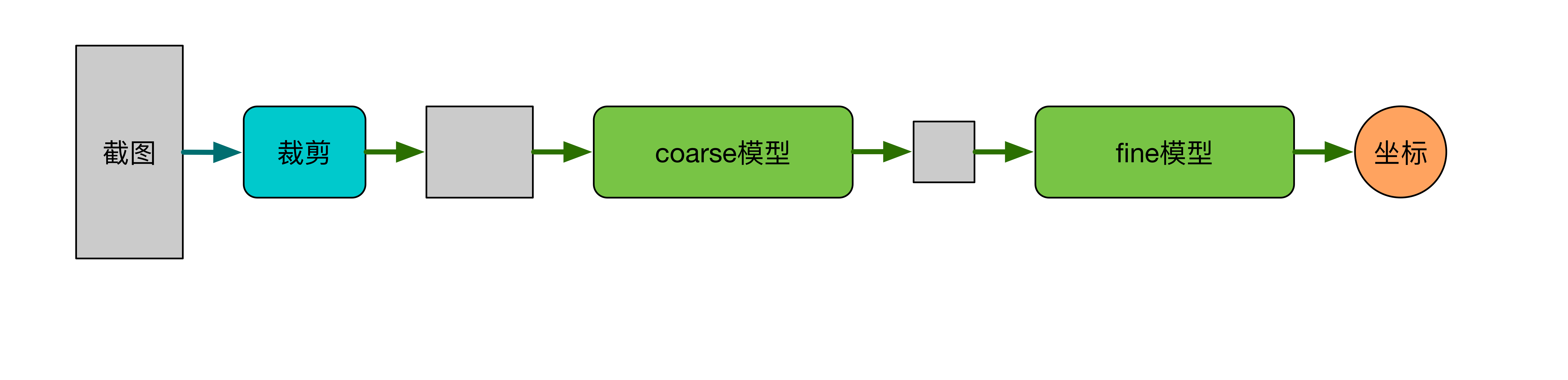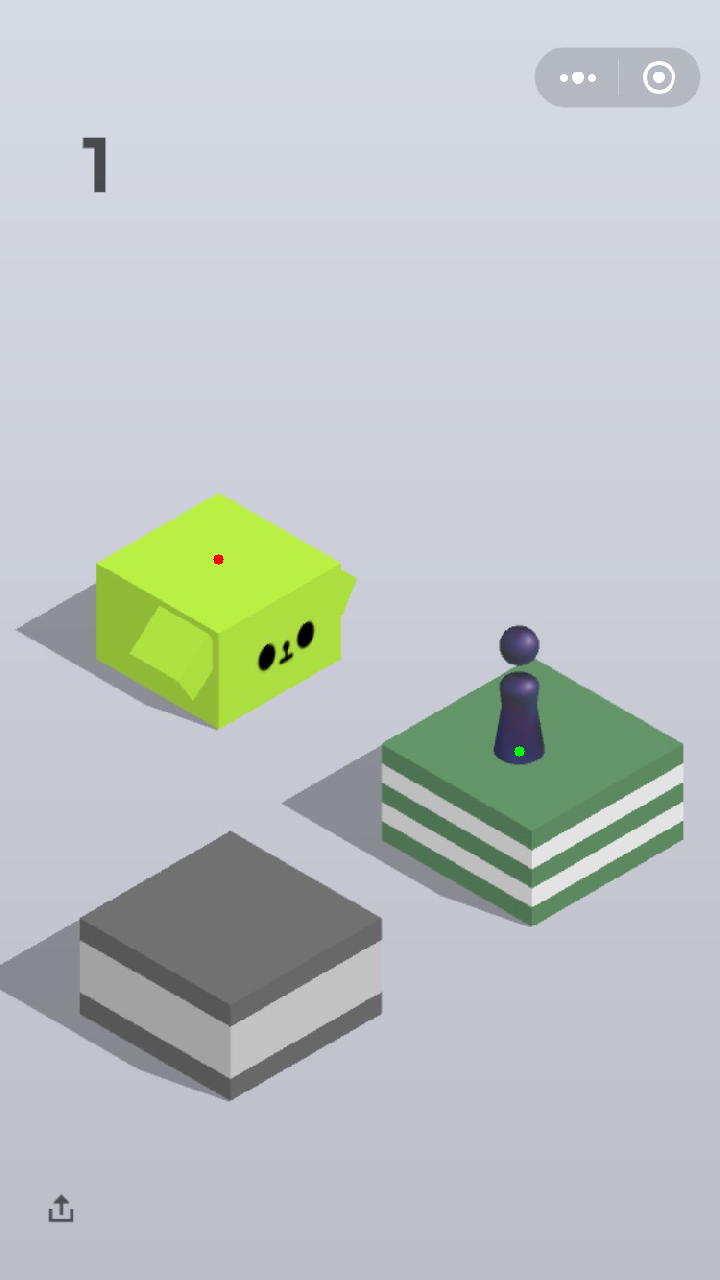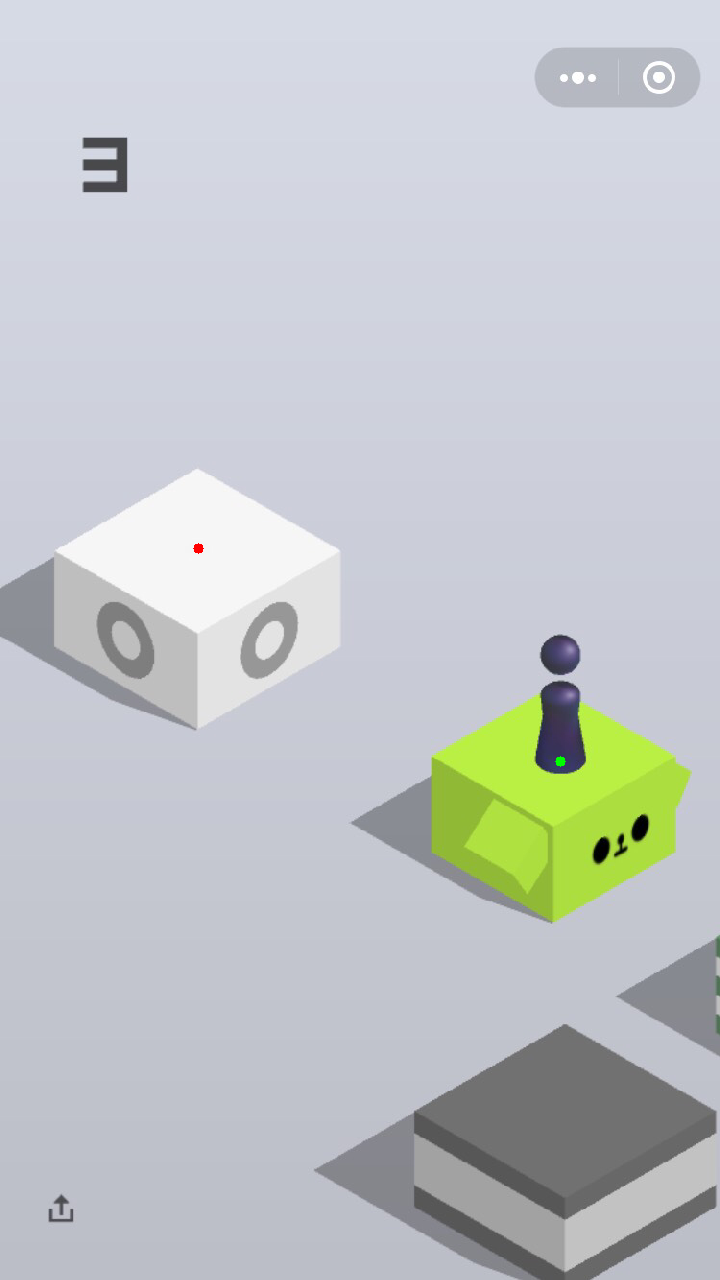Tags: